# How to use REGEXREPLACE Function in Google Sheets? (With Examples)

REGEXREPLACE function in google sheets is used to replace a part of a text string with a different text string using regular expression.

Regular expressions are sophisticated search patterns or character sequences that allow you to find specific patterns in a string. Once found, they can be replaced with any other text using the REGEXREPLACE function.

The REGEXREPLACE is one of the three regex functions in google sheets along with the REGEXEXTRACT and REGEXMATCH functions.

## What Does the REGEXREPLACE Function in Google Sheets Do?

The REGEXREPLACE function in google sheets simply replaces the part of the text string with a different text string. The REGEX function is called a regular function which matches a particular pattern and replaces it with different text.

## Syntax of the REGEXREPLACE Function in Google Sheets

=REGEXMATCH(text, reg_exp, replace_text)

In the above syntax,

• text – is the text or string that has to be replaced
• reg_exp – is the regular expression with a particular pattern. This expression matches part of text or string that has to be replaced
• replace_text – is the text that will be replaced with all the parts of text or string that match the reg_exp

### Application of the REGEXREPLACE Function in Google Sheets

When you want to replace a specific search string or pattern in multiple cells, the REGEXREPLACE function in google sheets can be very useful.

Below are some of the useful applications of the REGEXREPLACE function in google sheets,

• It can be used to replace or remove a specific letter, word, or phrase in different cells.
• It can be used to remove or replace all spaces in a text.
• It can be used to delete or replace all numerical values in a string.
• It can be used to remove or replace all URLs in a string.
• It can be used to remove HTML tags from a string.

There are many other ways also in which you can use the REGEXREPLACE function in Google sheets.

Let’s see in detail how the REGEXREPLACE function is used in the above application below,

### Using REGEXREPLACE Function in Goole Sheets to Replace or Remove a Letter, Word, or Phrase in a String

Here let’s see how to remove a specific letter, word, or phrase in a string using the REGEXREPLACE function in google sheets from the selected cell,

Example,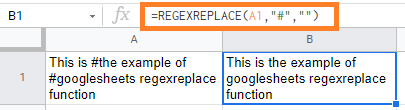In the above example, If we want to remove the hash symbol ‘#’ from the text in the A1 cell.

For this, we are using the REGEXREPLACE function as follows,

=REGEXREPLACE(A1, “#”, “”)

Here we are replacing the ‘#’ with nothing.

If we want to replace the string “example” with “sample” then we can use below REGEXREPLACE function,

=REGEXREPLACE(A1, “example”, “sample”)

Below is the result you would get,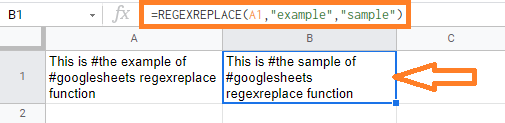We can also use the REGEXREPLACE function for removing and replacing more than one word in the cell.

Example: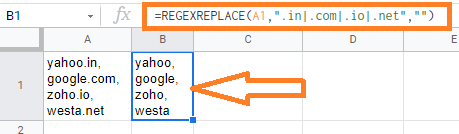In the above example,

We are removing multiple words like “.in, .com, .io, .net” from yahoo.in, google.com, zoho.in, westa.net with below formula,

=REGEXREPLACE(A1, “.in|.com|.io|.net”, “”)

The above expression is converted to Yahoo, Google, Zoho, Westa.

### Using REGEXREPLACE Function in Google Sheets to Remove All the Spaces From a String

Here let’s see how to remove the spaces in a string using the REGEXREPLACE function in google sheets from the selected cell,

Example,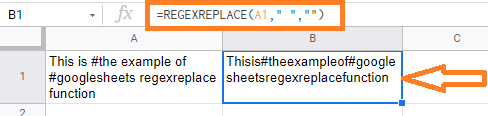In the above example, we are replacing the spaces with no space with the below expression,

=REGEXREPLACE(A1, ” “, “‘)

This expression removes all the spaces from the A1 cell text.

We could also replace the space with special characters like (!, @, #, \$, and so on) and also with any of the alphabets.

Example: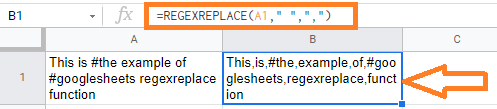In the above example,

=REGEXREPLACE(A1, ” “, “,”)

We are replacing the empty spaces with a comma – “,” in A1 cell text in the above expression.

### Using the REGEXREPLACE Function in Google Sheets to Remove or Replace All Numerical Values in a String

To replace or remove all the numbers from a cell, you need to use the “[0-9]” regex characters.

The square brackets are used to hold a set of characters. Since you want to match any number between 0 and 9, we use the 0-9 regular expression inside the square brackets.

This means ‘match any character that is between 0 and 9’.

Example: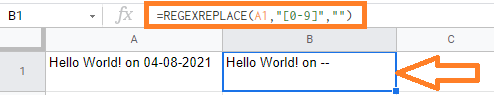In the above example,

=REGEXREPLACE(A1, “[0-9]”, “”)

We are removing the numbers from the A1 cell in the above expression.

Here, we can also remove or replace all the numbers which include decimal points.

Formula : =REGEXREPLACE(A1, “[0-9]*\.[0-9]|[0-9]”, “”)

In the above formula, we are using the “.” character preceded by the escape character “\” so that the “.” character is not mistaken for a regular expression.

The ‘*’ character denotes zero or more occurrences of a character or string, whereas the ‘+’ character denotes one or more occurrences of a character or string.

This ensures that the regular expression matches even numbers with no digits preceding the decimal point.

We added another [0-9] expression after the ‘|’ operator because we also want to consider cases where the number is an integer (with no decimal point at all).

Example: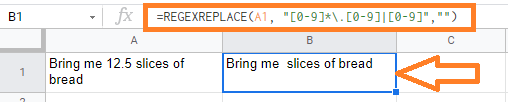In the above example, we are removing the decimal number 12.5.

### Using REGEXREPLACE function in Google Sheets to Remove or Replace Web URLs from the String

Here let’s see how to remove or replace the Web URLs in a string using the REGEXREPLACE function in google sheets from the selected cell.

To remove all the instances of web URL from the selected cell, we can use the below expression’s,

Formula:

• =REGEXREPLACE(A1, “www(.*)com”,””)
• =REGEXREPLACE(A1, “^www\.[a-zA-Z\.]+com”, “”)

This expression can be used to represent any number of characters. When we put this expression between the words ‘www’ and ‘com,’ it represents any string that begins with www and ends with com.

Example: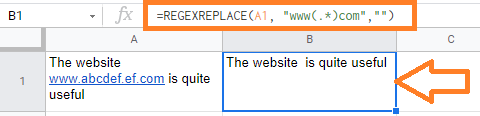In the above example, we are replacing the text prefixing “www” and ending with “com”.

### Using the REGEXREPLACE Function in Google Sheets to Remove HTML tags from a String

Here let’s see how to remove the HTML tags in a string using the REGEXREPLACE function in google sheets from the selected cell.

We can use the below formula to remove the HTML tags,

Formula:

=REGEXREPLACE(A1, “(\<([A-Za-z1-9]+)\>)|(\</([A-Za-z1-9]+)\>)”, “”)

The regular expression in the above formula can be divided into two parts,

• Opening tags – like <p> and <code>
• In the above formula , we are using the regular expression: (\<([A-Za-z1-9]+)\>). This matches all the instances starting with “<” and ends with “>”
• Closing tags – like </p> and </code>
• In the above formula , we are using the regular expression: (\</([A-Za-z1-9]+)\>). This matches all the instances starting with “</” and ends with “>”

The two expressions are separated with the ‘|’ symbol so that it matches either of the two sub-expression.

Example: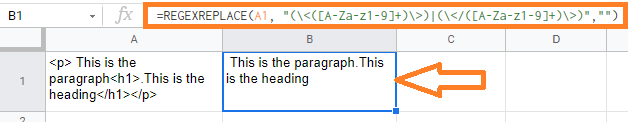In the above example, we are removing the HTML tags <p>,<h1>, </p>, </h1> in the A1 cell.